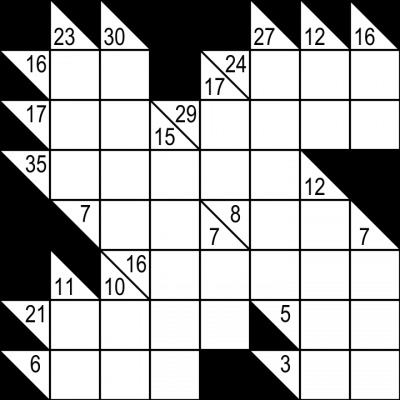# Difference between Associative and Commutative

Whenever you need to get the measurement of something, you are required to use numbers and equations. Numbers and solutions to complex equations has helped solve complex mathematical and algebraic problems. From the kitchen to the grocery store and everywhere in between, you need to use addition, subtraction, multiplication and division functions to compute how much you need to pay for goods and services. Practice has allowed us to perform these calculations effectively and efficiently. However, one can argue about the way you adapt to perform these calculations. Can these calculations be done in a different way? The answer lies within algebra where these functions and equations are defined.

In mathematics, an operation is said to be binary if it includes two quantities. More specifically, a binary operation is an operation between two elements from a set. The numbers in the set are known as operand. Most equations in mathematics are considered to be binary functions. Set theory, arithmetic operations, mathematical logics and linear algebra are all recognised as binary functions. These binary operations are defined depending on the two fundamental properties; Commutative and Associative.

If the result of the operation is not affected by the operand or number in the set then it is said to be a Commutative function, possessing Commutative properties. An Associative function, on the other hand, is a function where two or more occurrences of the operator do not affect the order of calculation or execution. Read on to find more differences between these two terms.

### Instructions

• 1

Associative

A function where the sequence of elements or order of execution does not affect the result of the operation is said to be Associative in nature. The example of an Associative function is given below:

A + (B + C) = (A + B) + C ⇒ 4 + (5 + 3) = (5 + 4) + 3 = 12

- Image courtesy: boundless.com• 2

Commutative

As discussed before, a Commutative operation is based on the fact the operands do no not affect the result of the operation. For example, if a binary operation x is applied on two elements called A and B and A x B = B x A then the operation is so be commutative in nature.

All multiplication and addition operations in algebra or mathematics are commutative operations. For example when you have any two numbers A and B, the result will be the same irrespective of the sequence of the elements, which means:

A + B = B + A and A x B = B x A
5 + 4 = 4 + 5 and 5 x 4 = 4 x 5

Subtraction and division functions can never be Commutative in nature.

- Image courtesy: golem.ph.utexas.edu•